Automation, Control and Intelligent Systems
Volume 3, Issue 2, April 2015, Pages: 26-30

Analysis and Research on Combination Feature Extraction Method of EEG Singnal

LI Jun-wei1, Jason Gu2, XIE Yun1

1Electronic & Information Engineering College, Henan University of Science and Technology, Luoyang Henan, China

2School of Biomedical Engineering, Dalhousie University, Halifax, Canada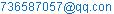(LI Jun-wei)(Jason Gu)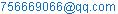(XIE Yun)

LI Jun-wei, Jason Gu, XIE Yun. Analysis and Research on Combination Feature Extraction Method of EEG Singnal. Automation, Control and Intelligent Systems. Vol. 3, No. 2, 2015, pp. 26-30. doi: 10.11648/j.acis.20150302.13

Abstract: EEG feature extraction problem is studied in this paper. EEG analysis is the core content of the Brain-computer interface technology research. How to effectively extract the reflect people's behavior intention characteristic from EEG signals, it's a hot spot in this neighborhood research. According to the characteristics of EEG signal, the single method of feature extraction can't describe the characteristics of the signal very well. So We have own designed experiment, and put forward a combination feature extraction method, which contains calculation the maximum Lyapunov exponent and use wavelet packet transform to calculate the rhythm average energy with wavelet energy entropy, then, the extract feature vector is inputted into the binary tree support vector machine (SVM) and the extreme learning machine (ELM), respectively. From the recognition result show that, when use the combination method of feature extraction to solve the problem of feature extraction and classification about this subject acquisition EEG, it's feasible and effective. At the same time, it also provides a new thought and method.

Keywords: EEG, The Maximum Lyapunov Index, Wavelet Packet Transform, ELM

1. Introduction

Today, The Brain-Computer Interface (BCI) technology has become a hot spot for researchers, it's a new control system that can help people to communicate information with the external environment, and it independent on the traditional way of using human brain to normal control the output of neural network and muscle tissue . BCI neeeds to transform the behavior intention of the EEG signal into the control signals of equipment, then achieve the corresponding task of people want to. So It can make the people whose  brain is normal but body is disabled through imagining movement to achieve direct communication with the outside world, such as controling computer, remote control cars, wheelchair, etc., thus it has a great value in using.

The EEG processing is the core technology content in the BCI technology, and how to correctly extracted the characteristics of people's behavior intention from the EEG is very critical in the using reasearch. Now most people use a single analysis method, such as AR model power spectrum estimation , wavelet transform , wavelet packet transform  and so on. Due to the complexity of EEG signal, making itself have the characteristics of randomness, non-stationary, nonlinear, diversity, rhythmicity, et al, only use one method can't describe the signal feature complete, resulting in the poor reliability on the next study. So the combination feature extraction method has received the people's attention. Now, according to the characteristics of EEG and combine with the research methods of EEG characteristics, make a variety of methods mutual fusion to solve  the problem of EEG feature extraction is a new train of thought.

This article mainly from the thought of uses the brain electrical to control the object ,such as mouse, toy car and so on, to do simple moving. Collected the four directions EEG signal of the brain imagining the object moving to the ‘Upward’ , ‘Downward’, ‘Liftward’, and ‘Rightward’. Then, combine with the rhythm energy and chaotic characteristics of EEG to explore a method of feature extraction. Firstly, do the preprocessing and selecte the channels. Secondly, putting forward a combined feature extraction method, which contain calculation the maximum Lyapunov exponent and use wavelet packet transform to calculate the rhythm average energy with wavelet energy entropy. Finaly, use the two methods of binary tree support vector machine (SVM) and extreme learning machine (ELM) to make recognition analysis about the combination characteristics. From the identify results, the combined feature extraction method has well effectiveness and practicability in the EEG feature extraction.

2. Data Acquisition and Preprocessing

2.1. The Experimental Data Acquisition

In this paper, according to the principle and characteristics of brain electrical produces, use the medical instrument NCERP series of EEG and evoked potentiometer 16 guide EEG acquisition device from Shanghai, the sampling frequency is 128 hz, choose three voluntary health subjects to collect the EEG signal of imagine object to do the four directions movement, respectively, notes for the letter of 'U', 'D', 'L', and 'R'. Acquisition process: subjects relaxation sitting in a comfortable chair, close eyes listen to the external command and imagine the corresponding movement, each direction do 40 times, the sampling time is 6s for one time, the first 1s as prepare, before after 1s as the end of the time, so the effective time is 4s, the total sampling points are 512. Complete the four directions as a set of experiment, the total data sets of 3 volunteers are 120.

2.2. Preprocessing

In the pretreatment is mainly research on signal filtering and channel selection. Combined with the characteristics and the effective research spectrum of EEG, choosing the band-pass filter of combine FFT with IFFT, selecting the mainly useful signal spectrum of 0.5-30 hz and removing interference. Then using the imagine upward direction of C3 channel as example, seeing the effect from the Fig. 1.Figure 1. The instance of the imagine upward movement of C3 channel.

In the Fig.1, a is the original figure, b is the spectrum diagram, c is the spectrum diagram after bandpass filter, d is the waveform diagram after IFFT transformation. Seeing from the result that after the bandpass filter, eliminating the power frequency interference, and some low frequency and high frequency noise, improve the signal-to-noise ratio.

According to domestic and international research experience, the signal energy wave will change as soon as the brain to imagine movement, but the change is not obvious in every channel , so in order to lighten the load of research, selecting the research channel. Assume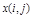is the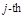point EEG data of the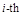test, then, the average electrical energy of the sample poin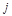in the total tes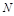is: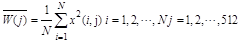(1)

when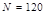, use the equation (1) to calculate the 16 electrode locations average energy for the four imagine directions, the average energy of each direction in each electrode is shown in Fig. 2.

From the Fig. 2. it is observed that average energy has significant overlaps and saltation in addition to the electrode locations of C3, C4, P3 and P4, and in this four points, the change of average energy is progressive, smooth. At the same time, from the brain anatomy, the central lobe and the parietal lobe are the feeling nervous centralis, so the four channels can be used as research object.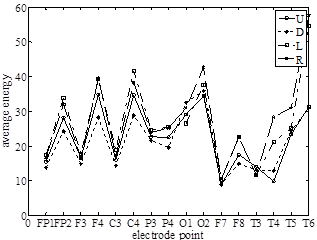Figure 2. the average energy of the four directions on the 16 electrode locations.

3. Feature Extraction

3.1. Calculat the Maximum Lyapunov Exponents Method

Lyapunov exponent is one of the major parameters to measure a chaotic sex. It can quantitatively describe the average rate of mutual convergence or divergence over time about two tracks on approximate initial state in the system phase space. Combine with the chaotic characteristic of EEG signals, in this article, choose the maximum Lyapunov exponent to describe the characteristics of eeg changes. Under the experience of the previous studies, adopt the minimum volume method to calculate the maximum Lyapunov exponent, and use the C-C algorithm to calculate the two parameters of embedding dimension and optimal delay time .

Let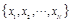is the time chaotic sequence, with the delay coordinate method, the phase space reconstruction is given by: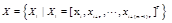(2)

Where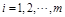,is the delay time,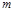is the embedding dimension,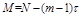is the points of phase space. The steps of algorithm are as follow:

Step1: Use the FFT transform to calculate the time series average period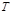, take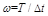is separation interval,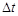is the sampling period of time sequence.

Step2: Use the C-C algorithm to calculate the embedding dimension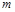and optimal delay time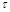.

Step3: Reconstruct the phase space by the embedding dimensionand optimal delay time.

Step4: Calculate the most near distance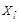of everypoint, define the shortest distance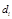is: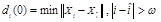(3)

Step5: Calculate the distance of the point, which close to the each phase space point, after thediscrete time step. The distance is: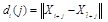(4)

Where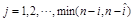.

Step6: Calculate the maximum Lyapunov exponent, it is defined as: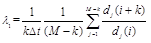(5)

The time-varying of the maximum Lyapunov exponent about the four imaginary movement directions of C3 channel are showing in the Fig.3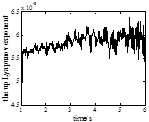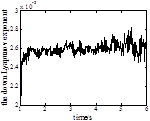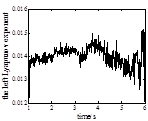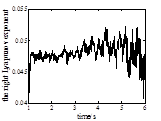Figure 3. The time-varying of the maximum Lyapunov exponenthere of C3 channel.

From the Fig.3, it is noted that each Imagine movement direction has clearly change in the the maximum Lyapunov exponent graph. After a great deal of experiments simulation, It is found that, in the C3 channel, the maximum Lyapunov exponent of upward imagine movement changes in the range of 0.0044 ~ 0.0048, the downward imagine movement changes in the range of 0.0021~0.0027, the leftward imagine movement changes in the range of 0.0121~0.0146, and the rightward imagine movement changes in the range of 0.0418~0.0501. Thus, it is also found that the chaos characteristic of the four imagine movement directions EEG signal has certain distinction in the C3 channel. Although there may be overlap part through large amounts of data test, it can still as the distinction between the four types of signals. So the the maximum Lyapunov exponent can be used to describe the feature of the four imagine movement EEG signal.

3.2. Wavelet Packet Transform Feature Extraction

The EEG signal consists of four kinds of rhythm wave, there will obviously shown a different rhythm transformation, as soon as the brain made any physiology. The wavelet packet transform is an improvement of the wavelet transform, it decomposes the signal in level and can focuse on all scope, According to these reasons, it can be used to extract different rhythms of EEG signal and overcome the shortcomings of traditional spectrum analysis.

Combine with the rhythm characteristic of EEG, choose the db6 wave to decompose the EEG singnal at 4 levels , getting four kinds of EEG rhythm wave, there are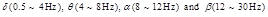, choose one trial as example, the change of four rhythm waves on the C3 channel are showing in the Fig.4.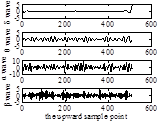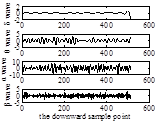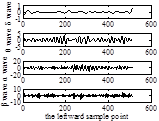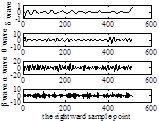Figure 4. The four kinds of rhythm waveform of C3 channel.

From the Fig.4, it is observed that the four rhythm waves have clearly change in the four Imagine movement directions, so the rhythm can be used to describe the EEG characteristic.

Then, claculate the average energy as the characteristics of each direction rhythm wave, the wavelet rhythm average energy is defined as:(6)

Where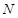is the total sample points,is the average energy of the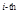rhythm wave. set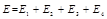, then, after normalized process, the normalized vector was become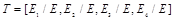.

Through the study of entropy, found that the wavelet packet energy entropy can also reflect the wave energy changes . So in this paper, increasing the solving of wavelet packet energy entropy, it is defined as: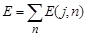(7)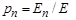(8)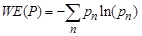(9)

Where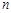is thesubspace in the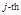wave layer,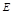is the total energy,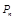is the signal energy distribution probability in the subspace.

Use the equation (6), (7), (8) and (9) to calculate the overall wavelet rhythm average energy and wavelet packet energy entropy of the total 120 samples in the four directions of C3 channel, they are shown in the Table 1.

Table 1. the four kinds of rhythm wave energy mean(mean ±standard deviation) and wavelet packet energy entropy of C3 channel.

 U D L R δwave 0.0058±0.0050 0.0069±0.0080 0.0034±0.0045 0.0050±0.0022 θ wave 0.1129±0.0297 0.1360±0.0321 0.0992±0.0216 0.1052±0.0443 αwave 0.7571±0.0434 0.7224±0.0695 0.7681±0.0333 0.7562±0.0442 β wave 0.1242±0.0283 0.1347±0.0440 0.1293±0.0165 0.1336±0.0215 energy entropy 0.7460 0.7106 0.7158 0.7436

From the Table 1, the energy change ofαwave is conform to the EEG characteristics of close eyes, it is the largest energy value among the four rhythm waves. From the energy entropy value, the complexit of the four imagine movement directions EEG signal have few difierence. So they can be used to describe the EEG characteristic.

3.3. Combination Feature Extraction Method of EEG Signal

According to the above statement, computing the maximum Lyapunov exponent as 4 dimensionalities characteristics, combined with the wavelet packet transform method of 20 dimensionalities characteristics, consisting of 24 dimensionalities signal combination characteristics. The combination characteristics vector of the upward imagine movement EEG signal are shown in Table 2.

Table 2. the combination characteristic value of the upward movement EEG.

 channel Lyapunov exponent δwave θ wave αwave β wave energy entropy C3 0.0041 0.0060 0.1332 0.7555 0.1409 0.7457 C4 0.0043 0.0052 0.1323 0.7415 0.1387 0.7142 P3 0.0042 0.0046 0.1311 0.7089 0.1322 0.7336 P4 0.0046 0.0042 0.1401 0.7033 0.1368 0.7425

4. Pattern Classification Verification

Based on the multiple classification problems, in consideration of the nonlinear features of EEG signal, select the support vector machine (SVM) and the extreme learning machine (ELM) to test whether the combined feature extraction method is effective for identification authentication or not, the conclusion is from the the recognition results.

The SVM is designed by the basis of the structure risk minimization combines with the statistical theory, through constructing the optimal hyperplane to realize the final minimum classification error of the unknown samples. When solve the multiple classification problems, it needs to construct multiple suitable SVM . In this article, use the binary tree support vector machine, the kernel function is radial basis function. The test steps: Firstly, use the datas of ‘U’ and ‘D’ to train the SVM1; Secondly, use the combination datas of ‘U’ and ‘D’ with ‘L’ to train the SVM2, Thirdly, using the combination datas of ‘U’, ‘D’, and ‘L’ with ‘R’ to train the SVM3, then, complete the train and obtained a multiple SVM classification, it is confirmed effective after done simulation test. When testing, start from the SVM3 classifier and then tested samples resulto until the symbols of decision functions is positive, the signal category is comfirmed at the end.

The ELM is a typical single hidden layer feedforward neural network, which has simple operation and effective characteristic . It only needs to set the number of hidden layer nodes and don't need to adjust the network weights of the input and hidden bias among the testing, and produces the unique optimal solution. Compared with the SVM classifier, in the classification problems, the ELM classifier don't need to build multiple classifier, it can be realized by setting a single neural network with multi-output votes. In this article, selecting the sigmoidal excitation function to identify the samples.

5. Results and Analysis

Selecting 120 samples of three volunteers at each imagine movement direction to do simulation test, choice 90 samples as training samples at random, the rest are testing samples. Use the two classifiers to identify the characteristic vector from the three feature extraction methods, respectively. The identify results are shown in the following table.

Table 3. The identify results of three feature extraction methods in the two classifiers(%).

 classifier U D L R SVM Lyapunov exponent 64.3 68.5 76.6 74.3 wavelet packet 73.4 70 74.6 73.3 combination feature 78.4 79.2 81.7 83.9 ELM Lyapunov exponent 68.3 70 76.8 75.2 wavelet packet 77.4 72.6 75 73.6 combination feature 80.4 81.6 87.6 83.3

Table 4. The average identify rate (%) and the training time (s) of the combination feature extraction method in the two classifiers.

 classifier combination feature method average identify rate training time SVM 80.8 17.265 ELM 83.225 13.168

From the Table 3 and Table 4, compared with the two method of the maximum Lyapunov exponent and the wavelet packet transform, the combination feature method has preferable identify resuls. Meanwhile, it also proves that the single method of feature extraction can't describe the characteristics of the EEG signal very well, while the combination feature method can do it and has good results. Besides that it also shown the advantage of choosing identify in solving multiple classification problem, compared with the SVM, the ELM has good quickness and accuracy features. The method of this paper can provide a new thought the brain-computer interface for online learnig.

6. Conclusion

Along with the advancing application value of the brain-computer interface, it is very important to find a effective feature extraction way to solve the EEG signal preoblem. In this paper, gives a thought of using the maximum Lyapunov exponent combines with the wavelet packet transform to extracting the characteristics of the imagine movement directions, at the same time, uses two difierent identifies to prove the combination feature method is reliability and accuracy. In addition to do these, in order to reduce the blindness and workload of research, the paper also gives a method to selecting research channels. In general, the thought of this article can provide a new inspire in the feature extraction of EEG signal.

Acknowledgment

The author would like to thank Drs. Jason Gu for providing the theoretical support, and also thank to XIE Yun for providing sample datas.

References

1. J. R. Wolpaw, N. Birbaumer, D. J. McFarland, G. Pfurtscheller, T. M. Vaughan, "Brain-Computer Interfaces for Communication and Control", Clinical Neurophisiology, vol. 113, pp. 767-791, 2002.
2. A. Subasi, M. K. Kiymikl, A. Alkan, E. Koklukaya, "Neural network classification of EEG signals by using AR with MLE preprocessing for epileptic seizure detection", Math Comput Appl, vol. 10(1), pp. 57-70, April 2005.
3. D. Cvetkovic, E. D. Ubeyli, I. Cosic, "Wavelet transform feature extraction from human PPG, ECG, and EEG signal responses to ELF PEMF exposures: A pilot study", Digital Signal Process Rev J, vol. 18(5), pp. 861-874, September 2008.
4. Yang Banghua, Liu Li, Zan Peng, Lu Wenyu, "Wavelet packet-based feature extraction for brain-computer interfaces", Lect. Notes Comput. Sci., vol. 6330 LNBI(PART 3) , pp.19-26, 2010.
5. Saha Anuradha, Konar Amita, Ralescu Anca, Nagar Atulya K., "EEG analysis for olfactory perceptual-ability measurement using a recurrent neural classifier", IEEE Trans. Human Mach. Syst., vol. 44(6), pp. 717-730, December, 2014.
6. F. Shayegh, S. Sadri, R. Amirfattahi, K. Ansari-Asl, "A model-based method for computation of correlation dimension, Lyapunov exponents and synchronization from depth-EEG signals", Comput. Methods Programs Biomed., vol. 113(1), pp. 323-337, 2014.
7. Yang Renhuan, Song Aiguo, Xu Baoguo, "Analysis of EEG basic rhythms based on discrete harmonic wavelet packet transform", Dongnan Daxue Xuebao, vol. 38(6), pp. 996-999, November 2008.
8. Sun Yuge, Ye Ning, Xu Xinhe, "The feature extraction and recognition of EEG based on wavelet entropy and distance", Chinese Contr. Decis. Conf., CCDC, 2008, pp. 4294-4298
9. Chen Shanshan, Meng Qingfang, Zhou Weidong, Yang Xinghai, "Seizure detection in clinical EEG based on multi-feature integration and SVM", Lect. Notes Comput. Sci., vol. 7996 LNAI, pp. 418-426, 2013.
10. Yuan Qi, Zhou Weidong, Li Shufang, Cai Dongmei, "Approach of EEG detection based on ELM and approximate entropy", Yi Qi Yi Biao Xue Bao, vol. 33(3), pp. 514-519, March 2012.

 Contents 1. 2. 2.1. 2.2. 3. 3.1. 3.2. 3.3. 4. 5. 6.
Article ToolsAbstractPDF(257K)